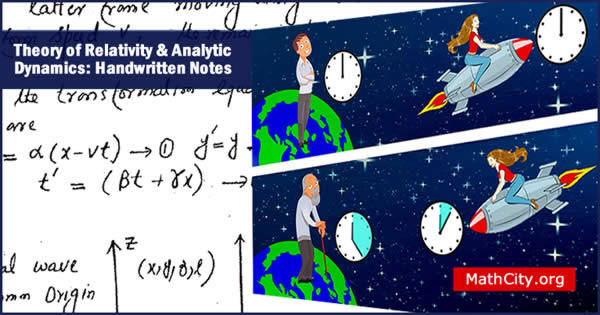# Theory of Relativity & Analytic Dynamics: Handwritten NotesTheory of Relativity and Analytic Dynamics is a subject that encompasses two distinct topics: relativity theory and analytic dynamics. Albert Einstein's theory of relativity defines the fundamental principles that control how moving objects behave in relation to one another and to the observer. The motion of objects as a result of forces and torques is the subject of analytical dynamics, a subfield of mechanics. It describes and forecasts the mobility of objects in space and time using mathematical techniques. In these notes

These are the notes of Prof. (Rtd) Asmat Ullah, Government College Sargodha, which is now University of Sargodha. Most of analytic dynamics topics are covered by these notes and very few topic of theory of relativity are given. But still these notes are helpful.

 Name Theory of Relativity & Analytic Dynamics: Handwritten Notes Prof.(Rtd) Asmat Ullah 132 pages PDF (see Software section for PDF Reader) 3.51 MB

#### Theory of Relativity

• Lorentz Transformation
• Effect of Lorentz Transformation on our Motion of Time and Space
• Relaitivity of Simultoncity
• Relativistic Law of Addtion of Velocities
• Minkowski Space
• World Time or Proper Time
• Mass and Momentum
• Momentum Vector
• The 4 Force
• The Kinetic Energy
• Transformation Equation for the Force Components
• Motion with Variable Proper Mass

#### Analytic Dynamics

• Rule of Cancellation of Dot
• System of n-particles
• Generalized co-ordinates
• Degree of Freedom
• Classification of Dynamical System
• Sclernomic or Pheonomic System
• Holonomic or Non-Holonomic System
• Conservative and Non- Conservative System
• D-Alembert's Principle
• Lagrange Equation of Motion
• The Equation related to the Lagranges Equation of Motion
• Equation of Motion of Particle in Space under Gravity
• Principle of Least Action
• Delafield Derivation
• Lagrange Equation for Non- Holonomic System
• Canonical or Conjugate Momentum
• Cyclic or Ignorable Co-ordinate
• Conservation of Momentum and Displacement of the System
• Hamiltonian Function and total Energy
• Hamiltonian Formulation of Mechanics
• Legendre Transformation
• Hamilton’s Equation of Motion
• Routh’s Procedure
• Equation of Hamilton’s Equation to central Force problem
• Derivation of Hamiltonian Equations from Variational Principle
• Hamilton’s Principle of Least Action
• Different forms of Principle of Least Action
• Canonical Terms Formation or Contact Transformation
• Examples of Canonical Transformation
• More General Form of Equation A
• Application to Harmonic Oscillator
• Hamiltonian Formulism
• Orthogonal Point Transformation
• The Poincare Theorem
• Lagrange Bracket
• The Poisson Bracket
• Poisson Brackets of Arbitrary Function
• Equation of Motion and Poisson Brackets
• Infinitesimal Contact Transformation
• Infinitesimal Canonical Transformation and Poisson Brackets
• Jacobi Identity
• Hamilton Jacobi Theorem
• Application of Hamilton Jacobi Theory
• Stockel’s Theorem
• Gauge Vitiate
• Nother's Theorem
• Poisson's Theorem

• notes/theory-of-relativity-and-analytic-dynamics